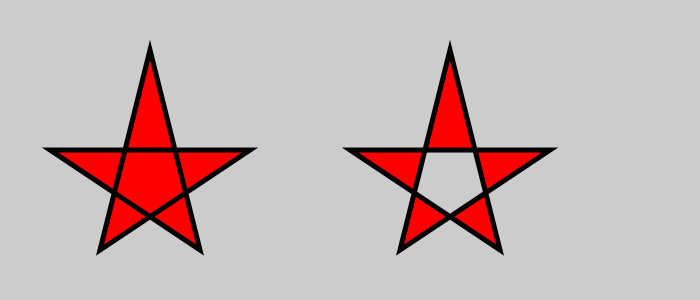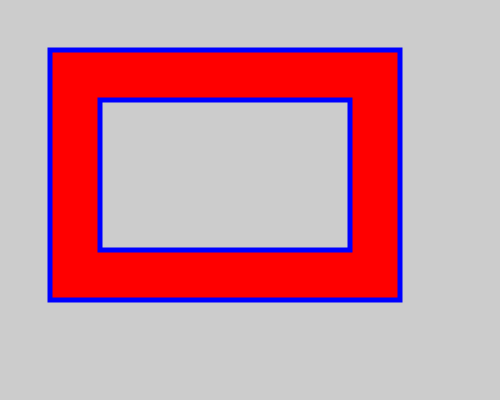# Complex paths in generativepy

Martin McBride, 2022-01-05
Tags generativepy tutorial complex path hole self intersection
Categories generativepy generativepy tutorialThis tutorial shows how to complex paths in generativepy. It follows on from the composite path tutorial.

A complex path is a single path that either:

• Encloses more than one area.
• Contains more than one subpath.
• Or both.

The main difference between a complex path and multiple simple paths is that the complex path is treated as one path when it is filled. This means that, depending on the fill rule selected, various parts of the shape will be filled or unfilled.

## Complex polygons

A complex polygon can enclose more than one area, if it is self-intersecting. A well known example is a star-polygon:

from generativepy.drawing import make_image, setup, EVEN_ODD
from generativepy.color import Color
from generativepy.geometry import Polygon
import math

def draw(ctx, pixel_width, pixel_height, frame_no, frame_count):
setup(ctx, pixel_width, pixel_width, background=Color(0.8))

black = Color(0)
red = Color('red')

Polygon(ctx).of_points([(150, 50),
(100, 250),
(250, 150),
(50, 150),
(200, 250),
]).fill(red).stroke(black, 5)

Polygon(ctx).of_points([(450, 50),
(400, 250),
(550, 150),
(350, 150),
(500, 250),
]).fill(red, fill_rule=EVEN_ODD).stroke(black, 5)

make_image("complex-polygon.png", draw, 700, 300)


This code is available on github in tutorial/shapes/complex-polygon.py.

Here is the resulting image:The code simply draws two polygons, each with 5 points. Due to the order the points are defined in the of_points list, the shape is drawn as a star shape rather than a simple convex pentagon.

In the first case we use the WINDING fill rule (the default) which causes the whole shape to be filled.

In the second case we specify the EVEN_ODD fill rule, which causes the central region of the star shape to be unfilled.

## Multiple subpaths

This code draws two rectangles, however it creates them as two separate subpaths within the same path:

from generativepy.drawing import make_image, setup, EVEN_ODD
from generativepy.color import Color
from generativepy.geometry import Line, Rectangle

def draw(ctx, pixel_width, pixel_height, frame_no, frame_count):
setup(ctx, pixel_width, pixel_width, background=Color(0.8))

black = Color(0)

Rectangle(ctx).of_corner_size((100, 100), 250, 150).as_sub_path()\
.fill(Color('red'), fill_rule=EVEN_ODD)\
.stroke(Color('blue'), 5)

make_image("complex-subpaths.png", draw, 500, 400)


This code is available on github in tutorial/shapes/complex-subpaths.py.

Here is the resulting image:We create the first Rectangle object, but instead of filling or stroking it, we use add() to add it to the current path (similar to creating a composite path).

For the second Rectangle, we call as_sub_path() to add the item as a subpath within the existing path.

When we fill and stroke the item, both rectangles will be drawn because they are both part of the path. Due to the EVEN_ODD fill rule, the inner rectangle is unfilled.

If you found this article useful, you might be interested in the book Computer Graphics in Python or other books by the same author.

#### Popular tags

2d arrays abstract data type alignment and angle animation arange arc array arrays behavioural pattern bezier curve built-in function callable object chain circle classes clipping close closure cmyk colour combinations comparison operator comprehension context context manager conversion count creational pattern data science data types design pattern device space dictionary drawing duck typing efficiency ellipse else encryption enumerate fill filter font font style for loop function function composition function plot functools game development generativepy tutorial generator geometry gif global variable gradient greyscale higher order function hsl html image image processing imagesurface immutable object in operator index inner function input installing iter iterable iterator itertools join l system lambda function len line linear gradient linspace list list comprehension logical operator lru_cache magic method mandelbrot mandelbrot set map matplotlib monad mutability named parameter numeric python numpy object open operator optimisation optional parameter or pandas partial application path pattern permutations polygon positional parameter print pure function python standard library radial gradient range recipes rectangle recursion reduce repeat rgb rotation roundrect scaling scipy sector segment sequence setup shape singleton slice slicing sound spirograph sprite square str stream string stroke structural pattern subpath symmetric encryption template text text metrics tinkerbell fractal transform translation transparency triangle truthy value tuple turtle unpacking user space vectorisation webserver website while loop zip